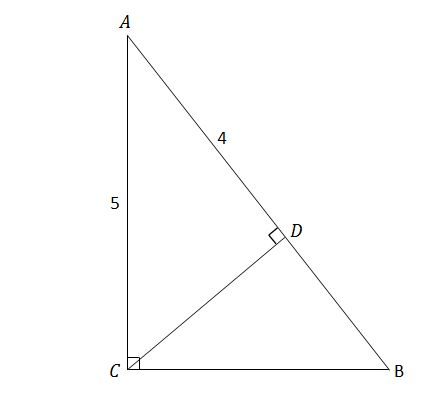# No angle allowed!

Geometry Level 2Triangle $ABC$ is a right triangle with a side length $\left\lvert\overline{AC}\right\rvert=5, \angle C = 90^\circ$ and the measures of $\angle A$ and $\angle B$ unknown. If $D$ is a point on $\overline{AB}$ such that $\angle ADC = 90^\circ$ and $\left\lvert\overline{AD}\right\rvert=4,$ what is $\left\lvert\overline{BC}\right\rvert?$

×